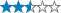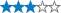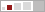<

## JJJ Light up the Ridge

A cache by BoazRuthFields and DAandDI Message this owner
Hidden : 08/29/2018
Difficulty:Terrain:Size:(small)

#### Watch

How Geocaching WorksPlease note Use of geocaching.com services is subject to the terms and conditions in our disclaimer.

### Geocache Description:Hobby Park Light Up the Ridge Multi
Welcome to the Mulder's Drift region a really lovely nature area. This puzzle is one of many created for the 2018 Jozi Jol Mega. Solve the puzzle and go on a meandering hike through the Suikerbos Proteas to retrieve the cache. Day hikers, mountain bikes and off road vehicle are welcome at Hobby Park. An entrance fee is payable at the gate. Even with an off road vehicle a short hike to the cache is required.

This a 5 stage multi for the adventurous. It run along the rocky ridge of the northen border of the Hobby Park. From this ridge you see the whole Hobby Park below with its Protea trees, tracks and picnic spots. The cache is hidden away from the tracks and paths of the park. Please take care while solving this cache as the rock crevases and long summer grass may hide the local fauna.(snakes, spiders, ticks etc). No climbing over and above the actual hike is required to retrieve any of the caches.

You will need to solve each stage in sequence to get the co-ordinates of the next stage. Make sure you have at least 2 well charged AA batteries with you to help solve each stage.

STAGE 2 CO-ORDINATES:

S26 04. { R1+B1 }{ R1+B1+W1 }{ 2(G1)+Y1+W1 }

E27 49.{ (G1x R1)/B1 }{R1+B1-W1-Y1 }{ (B1x W1)+G1-W1 }

STAGE 3 CO-ORDINATES:

S26 04. { R2+B2-Y2 }{ (R2xB2) - (W2/Y2)/G2 }{ Y2-G2 }

E27 49.{ R2 }{ R2 +B2 }{ B2 }

STAGE 4 CO-ORDINATES:

S26 04. { R3+B3+W3 }{ ( Y3 x W3) / (B3 x G3) }{ B3 x G3 + W3 - R3 }

E27 49. { Y3 x R3 }{ ( ( R3 + B3)G3)-W3-Y3 }{ G3 x B3 + W3 }

FINAL STAGE CO-ORDINATES:

S26 04. { R(1+2+3+4)+Y(1+2+3+4) - B(1+2+3+4)}{ B(1+2+3+4)) - (G(1+2+3+4) / R(1+2+3+4)) }{ Y(1+2+3+4) - W(1+2+3+4)}

E27 49. { 2(Y(1+2+3+4)) - B(1+2+3+4) }{ B(1+2+3+4) / (G(1+2+3+4) - W(1+2+3+4)) }{ G(1+2+3+4)) + R(1+2+3+4) - B (1+2+3+4)) }